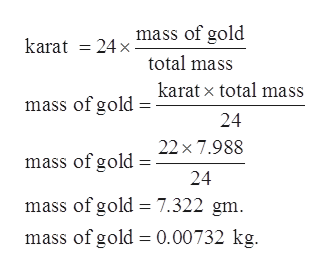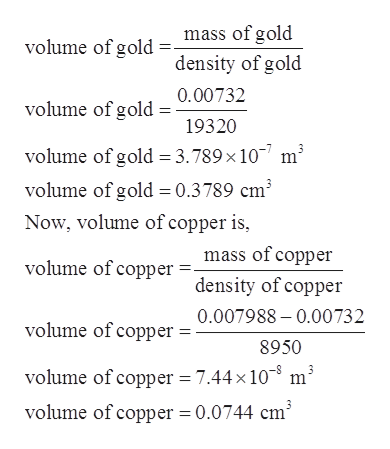# The British gold sovereign coin is an alloy of gold and copper having a total mass of 7.988 g, and is 22-karat gold. (a) Find the mass of gold in the sovereign in kilograms using the fact that the number of karats = 24 x (mass of gold)/(total mass). (b) Calculate the volumes of gold and copper, respectively, used to manufacture the coin. (c) Calculate the density of the British sovereign coin.

Question
12 views

The British gold sovereign coin is an alloy of gold and copper having a total mass of 7.988 g, and is 22-karat gold. (a) Find the mass of gold in the sovereign in kilograms using the fact that the number of karats = 24 x (mass of gold)/(total mass). (b) Calculate the volumes of gold and copper, respectively, used to manufacture the coin. (c) Calculate the density of the British sovereign coin.

check_circle

Step 1

Given:

Total mass of British sovereign coin = 7.988 g

Gold karat in coin = 22 karat.

Step 2

Part a)

The mass of gold is given as,help_outlineImage Transcriptionclosemass of gold karat = 24 x- total mass karat x total mass mass of gold 24 22 x 7.988 mass of gold : 24 mass of gold = 7.322 gm. mass of gold = 0.00732 kg. fullscreen
Step 3

Part b)

Density of copper = 8.95 g/cm3. = 8950 kg/m3.

Density of gold = 19.32 g/cm3. = 19320 kg/m3...help_outlineImage Transcriptionclosemass of gold volume of gold = density of gold 0.00732 volume of gold 19320 volume of gold = 3.789×10- m³ volume of gold = 0.3789 cm³ Now, volume of copper is, mass of copper volume of copper density of copper 0.007988 – 0.00732 volume of copper : 8950 volume of copper = 7.44 x 10-³ m³ volume of copper = 0.0744 cm fullscreen

### Want to see the full answer?

See Solution

#### Want to see this answer and more?

Solutions are written by subject experts who are available 24/7. Questions are typically answered within 1 hour.*

See Solution
*Response times may vary by subject and question.
Tagged in

### Physics